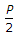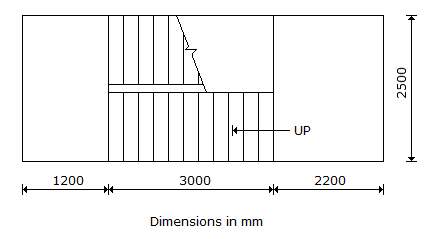# Civil Engineering - GATE Exam Questions

Exercise : GATE Exam Questions - Section 4
46.
A weir on a permeable foundation with downstream sheet pile is shown in the figure below. The exit gradient as per Khosla's method is1 in 6.0
1 in 5.0
1 in 3.4
1 in 2.5
Explanation:
No answer description is available. Let's discuss.

47.
Which one of the following set of values give the minimum clear cover (in mm) for the main reinforcements in the slab, beam, column and footing, respectively, according to IS : 456-1978 ?
20, 25, 30, 75
5, 15, 25, 50
15, 25, 40, 75
none of the above
Explanation:
No answer description is available. Let's discuss.

48.
Two flow patterns are represented by their stream functions ψ1 and ψ2 as given below:
ψ1 = x2 + y2, ψ2 = 2xy
If these two patterns are superimposed on one another, the resulting streamline pattern can be represented by one of the following :
A family of parallel straight lines
A family of circles
A family of parabolas
A family of hyperbolas
Explanation:
No answer description is available. Let's discuss.

49.
The forces in members 'a, b, c' in the truss shown are, respectively
P,, 0, P, 0
P, P, P,, 0